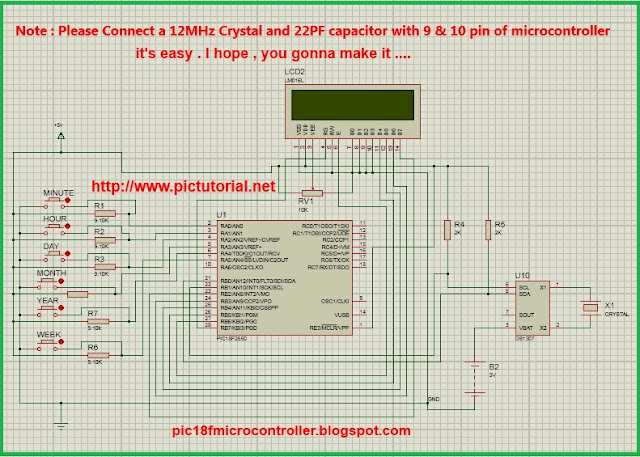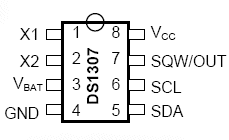# Learn Embedded Programming [Step by Step]

## Digital Clock [Real Time] Using DS1307 and PIC Microcontroller in Proteus

This tutorial contains description of microcontroller based digital clock and DS1307. We know microcontroller is a computer and specific time required to complete each task. So we cannot get real time and that's why we need a real-time clock IC. We will use DS1307 IC in this tutorial.Digital Clock [Real Time] Using DS1307 and PIC Microcontroller [step by step ]

## DS1307 IC:

DS1307 is a real-time clock IC which basically works with low power and returns BCD(Binary Coded Decimal)  number. We will describe later about BCD.
Through this IC we can get year, month, date, hour, minute and second. The months, days, second can be incremented automatically and It can operate either in 24-hour format or 12-hour format with AM/ PM.DS1307 has built-in power sensing circuit which can backup the IC automatically during the failure of power supply.Crystal (1000KHz)  is required to operate this IC and don't need any external capacitor connection.DS1307
At this stage, we have got basic information about DS1307. In this tutorial, we need to use I2C communication with Microcontroller. Description of I2C communication is given below.

### I2C Communication with Microcontroller using MikroC

The I2C(I Squared C) communication means "  Inter-Integrated Circuit " communication. A microcontroller can communicate with low power peripherals through I2C.To make a successful I2C communication we just need two ends or pins[ SCL and SDA ].Here SCL for clock selection and SDA for data. Both SCL and SDA are Open Drain drives that means the chip can drive it's low output and cannot drive the high output. It 's required to connect two pull up resistors in such way that one end of both resistors with +5v and others end with SCL, SDA respectively so that the higher output can be driven. The pull-up resistors value will be according to the I2C bus frequency.We are using 2k Ohm pull up resistor in this Tutorial.

#### MikroC Library for I2C :

• void I2C1_Init(const unsigned long clock); this function is needed to initialize and returns nothing .We have to provide frequency as it's arguments .    Example  : I2C1_Init(100000);
• unsigned short I2C1_Start(void) ; this function is needed to start communication .                  Example I2C1_Start();
• void I2C1_Repeated_Start(void); for starting again .
• unsigned short I2C1_Is_Idle(void);
Returns 1 if I²C bus is free, otherwise returns 0.

•  unsigned short I2C1_Rd(unsigned short ack); Returns one byte from the slave.
• unsigned short I2C1_Wr(unsigned short data_); Sends data byte (parameter `data`) via I²C bus.

• void I2C1_Stop(void); To stop the signal .

### The Time Keeper Register  of DS1307:#### The Time Keeper Register  of DS1307

Here we can see the address of each function ,data bits and data range .First we have to initialize the I2C using 10kHz frequency and start the communication using start function .Now if we like to write , it's required to send out 0xD0 [D0=0x68+0]through write function so that the IC can be enabled for writing to us.Instructions given below :
```I2C1_Wr(0xD0); [D0=0x68+0]
```
During reading the data comes from DS1307 , we need to send out 0xD1 [D1=0x68+1]through write functiono that the IC can be enabled for reading to us .Instructions given in below :
I2C1_Wr(0xD1);[D1=0x68+1]
```data = I2C1_Rd(0);
```
and it is a user defined function. In "Readdata(0);" function we will write 0 as
argument and the 0 means address of Seconds Register.This function returns
short type data in BCD . I this way we can read data from each address .An
important thing needed to notice that bit 6 of hour register is defined as the
24-hour or 12-hour mode selection bit.If we select 1 the bit 5 of hour used for
representing AM/PM and if we select 0 the bit 5 used for representing hour .

### BCD Number:

BCD number means Binary Coded Decimal number where each binary number is in 4bit .If we consider a decimal number 21 ,the BCD conversion will be 00100001 .Here blue represents the 2 and red represents 1. In that way we get BCD .Now the question is how can we get character from BCD  ?Cause if we like to show in LCD , we need character .Look at the code given below :

### First Char from BCD

char firstcharofbcd(char bcddata)
{
char tireturn;

tireturn= (bcddata >> 4) +0x30; Here we are shifting 4 bits to right hand side .

return tireturn;
}
If we consider 21 we can get 2 in character format .Because in short format data we added 0x30 , which is  character '0' .So the sum of '0'+0010 = '2' .

### Second Char from BCD

char secondcharofbcd(char bcddata)
{
char toret;
toret=(bcddata & 0x0F) + 0x30;

} .
0x0F means '00001111' .We know in an AND gate if any input is low or 0 and the gate output is 0.If all input is 1 and we can get output 1.So by applying AND operation between 0x0F and BCD , we can make first  0000 bit as 0 .Look at the example given below :

00100001
00001111 &
-------------
00000001= 1

I think we get all required basic information .Now lets create a project in Proteus .

### Proteus Project :

Pick all the required parts and Complete the circuit as given below :

### Note : In practical , you have to connect 12MHz crystal between pin9 and pin10 . Also remember that 1 & 20 will be connected with VDD and 8 & 19 will be connected with GND .Digital Clock [Real Time] Using DS1307 and PIC Microcontroller [step by step ]

Now we need the .hex file .So lets Create a project in MikroC .Please follow the steps given below :

## MikroC Code :

///////////////////////////////////////////////////////////////////////////////////////////////////////////////////////////////////////////////////////////////////

#### Source Code :

``````

sbit LCD_RS at RB7_bit;

sbit LCD_EN at RB6_bit;

sbit LCD_D5 at RB4_bit;

sbit LCD_D4 at RB5_bit;

sbit LCD_D6 at RB3_bit;

sbit LCD_RS_Direction at TRISB7_bit;

sbit LCD_D7 at RB2_bit;

sbit LCD_EN_Direction at TRISB6_bit;

sbit LCD_D5_Direction at TRISB4_bit;

sbit LCD_D4_Direction at TRISB5_bit;

sbit LCD_D6_Direction at TRISB3_bit;

I2C1_Wr(0xD0);

sbit LCD_D7_Direction at TRISB2_bit;

}

short getinp;

I2C1_Start();

I2C1_Repeated_Start();

I2C1_Wr(0xD1);

I2C1_Stop();

getinp=I2C1_Rd(0);
return getinp;

I2C1_Wr(writedat);

{

I2C1_Start();

I2C1_Wr(0xD0);

char firstcharofbcd(char bcddata)

I2C1_Stop();

}
{

char tireturn;

tireturn= (bcddata >> 4) +0x30;

return tireturn;
}

{

char secondcharofbcd(char bcddata)
char toret;

short wk;

toret=(bcddata & 0x0F) + 0x30;
}

char time[] = "  :  :    ";

char date[] = "  /  /         ";

short inc,in;
short chk;

short hr;

short sec;
short mint;

short h, ht;

char wkd;

short day;
short mon;

TRISA = 0x3F;
short yr;

void main() {

I2C1_Init(100000); //DS1307 I2C is running at 100KHz

inc=0;

CMCON = 0x07;  // To turn off comparators

ADCON1 = 0x0F; // To turn off analog to digital converters

Lcd_Init();            // Initialize LCD

Lcd_Cmd(_LCD_CLEAR);        // Clear display

Lcd_Cmd(_LCD_CURSOR_OFF);     // Cursor off

Lcd_out(1,1,"Time:");
while(1){

Finally i make it manually , you can see here .Thank You !!

h=hr; // for 12 hour format

// here means 00100000 in binary and we can get a signal in change of AM/PM

/* I dont know why 12hour format is not working .I worked hard but it didnot work .

*/

///////////////////////////////////////

chk=1;  }

h= Bcd2Dec(h);

if(h-12>=0){

ht=12 ;

if(h-12==0) {
chk=1;  }

chk=1;  }

if(h-12==1) {
ht=1 ;

ht=3 ;
if(h-12==2) {
ht=2 ;

chk=1;  }
if(h-12==3) {

chk=1;  }
chk=1;  }
if(h-12==4) {

if(h-12==5) {
ht=4 ;
ht=5 ;

if(h-12==6) {

chk=1;  }
ht=6;

if(h-12==7) {

chk=1;  }
ht=7;

chk=1;  }
chk=1;  }
if(h-12==8) {

if(h-12==9) {
ht=8;

ht=9 ;

chk=1;  }

if(h-12==10) {
ht=10;

if(h-12==11) {

ht=11 ;

} else{

chk=1;  }

chk=0;

if(h==0){
ht=12;

chk=0;

}

else{

ht=h;

///////////////////////////////////////////////////////////////////

}
}

h=Dec2Bcd(ht);

date = firstcharofbcd(day);

time = firstcharofbcd(h);

time = firstcharofbcd(mint);

time =secondcharofbcd(h);

time = firstcharofbcd(sec);

time = secondcharofbcd(mint);

date ='n';

time = secondcharofbcd(sec);

date = secondcharofbcd(day);

date = secondcharofbcd(mon);

date = firstcharofbcd(mon);
date = firstcharofbcd(yr);

switch(wkd){

date = secondcharofbcd(yr);
wkd=secondcharofbcd(wk);

date ='u';
case '1':
date ='S';

date ='u';

date ='d';

date ='y';

date ='a';
break;

date ='o';

case '2':

date ='M';

date ='a';

date ='n';

date ='d';

date ='T';

date ='y';
break;

date ='u';
case '3':
date ='e';

date ='d';

date ='y';

date ='a';
break;

date ='e';

case '4':

date ='W';

date ='a';

date ='d';

date ='d';

date ='T';

date ='y';
break;

date ='d';
case '5':
date ='h';

date ='d';

date ='a';

date ='y';

break;

date ='F';

case '6':

date ='i';

date ='r';

date ='a';

date ='d';
date ='y';

date ='S';

break;

case '7':

date ='a';

if(mint>59){

date ='t';
date ='a';

date ='y';

break;
}

time = 'P';

if(chk)
{

else

time = 'M';
}
{

time = 'M';

time = 'A';
}

Lcd_out(1, 6, time);

inc++;

Lcd_out(2, 1, date);
if(PORTA.F0==0){

delay_ms(300);
mint=Bcd2Dec(mint);

mint=0;
mint=mint+inc;
}

mint=Dec2Bcd(mint);

writedata(1,mint);

if(PORTA.F1==0){

}

delay_ms(300);

inc++;

inc=Bcd2Dec(h);
if(inc>23){

writedata(2,h);

inc=0;
}

h=Dec2Bcd(inc);
}

//////

inc=0;

if(PORTA.F2==0){

}          else if(mon==1 | mon==3 | mon==5 | mon==7 | mon==8 | mon==10| mon==12){
delay_ms(300);
in=Bcd2Dec(wk);

if(in>7){
in++;
in=1;

wk= Dec2Bcd(in);

}

///////

writedata(3,wk);

inc=Bcd2Dec(h);

inc++;

if(yr%4==0){

yr=Bcd2Dec(yr);

if(mon==2){

mon=Bcd2Dec(mon);

if(inc>31){

if(inc>29){
inc=1;

inc=1;
}

inc=1;

}

if(inc>30){

}else{

inc=1;
}

}

}else{

if(mon==2) {

mon=Bcd2Dec(mon);
if(inc>28){

else if(mon==1 | mon==3 | mon==5 | mon==7 | mon==8 | mon==10| mon==12){

inc=1;
}

if(inc>31){
}

if(PORTA.F4==0){

}

}else{

if(inc>30){

inc=1;
}

day=Dec2Bcd(inc);

}

}

if(PORTA.F3==0){

writedata(4,day);
}
inc=0;

}
inc++;
delay_ms(300);

inc=0;
if(inc>12){

}

mon=Bcd2Dec(mon);
mon=mon+inc;

mon=1;
if(mon>12){

inc++;

mon=Dec2Bcd(mon);
writedata(5,mon);

}
}
inc=0;

delay_ms(300);

if(inc>59){

yr=Bcd2Dec(yr);

inc=0;
}

if(yr>59){

yr=yr+inc;
yr=1;

writedata(6,yr);
}
yr=Dec2Bcd(yr);

if(PORTA.F5==0){
}

delay_ms(300);
//////

in=Bcd2Dec(wk);

wk= Dec2Bcd(in);

in++;

if(in>7){
in=1;

}
writedata(3,wk);

}
///////

}

``````

Compile Source Code

Load hex file to the microcontroller .

## Thank You!

Ain't getting any visitors!

## Featured Post

### Bluetooth Based Temperature Meter Project using Microcontroller and MikroC USART Terminal## Tags

: (1) 18F2550 (1) 36KHz (3) and (1) arduino (1) Based (1) battery (1) Bipolar (1) Blinking (1) blinks (1) Bluetooth (1) button (1) circuit (1) clock (1) control (1) Db9 (1) DC Motor (2) digital (2) display (2) DS1307 (1) electronic (1) flash (1) flashing (1) HC-06 (1) home (1) how (1) How to (10) i2c tutorial (1) in (1) indicator (1) interface (8) interfacing (3) Interrupt (3) Introduction (1) IR Receiver (4) key pad (1) keyboard (1) keypad (1) lavel (1) Lcd 16x2 (2) lcd 2x16 (2) led (1) lm35 (2) LPG (1) machine (1) make (1) making (1) matrix (1) max232 (1) meter (2) microchip (4) microchips (3) mikroC (5) musical (1) NEC Protocol (4) pcb (5) PIC (3) pic controller (11) pic proteus (1) Pic Tutorial (12) pic18 (2) pic18f2550 (11) picRFモジュール (1) proteus (6) push (1) push button (1) PWM (1) real (1) Rs 232 (1) Rs232 (1) scroll (1) scrolling (1) Serial Port (1) simulation (2) step by step (7) step bystep (1) text (2) time (1) timer (4) timer0 (4) tone (1) tutorial (2) Unipolar (1) USB (1) usb 1.0 (1) USB HID (1) using (9) voltmeter (1) voting (1) with (2) work (1)

## Live Traffic Feed

Live Traffic Feed
Visitor Tracking